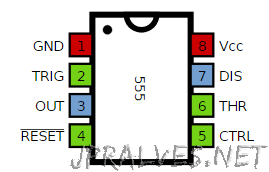Today we will build a circuit that is a timer.

The IC 555 was used. This was set to Mono-stable mode. When the button is pressed the time starts and the LED lights up. After a while it goes off.

To calculate the time the LED is lit the following formula is used: t = 1.1 * C * R where C is the value of capacitor C2 in Farads and R is the value of Resistance RV1 in Ohms. The time is in seconds.

If we want the time to be about 60 seconds, given that the capacitor used in the circuit is 100uF (0.0001F) the formula to use is the following R = 60 / (0.0001 * 1.1), R = 546000 Ohms.

## Schematic## Bill of materials (BOM)

Circuit:

• 1x 10K Ohms Resistor (R1)
• 1x 470 Ohms Resistor (R2)
• 1x 1M Ohms Variable Resistor (RV1)
• 1x IC NE555
• 1x 5mm Red LED (D1)
• 1x 100nF Ceramic Capacitor (C1)
• 1x 100uF Electrolytic Capacitor (C2)
• 1x SPST Button (SW1)

## IC/Components Pin-out